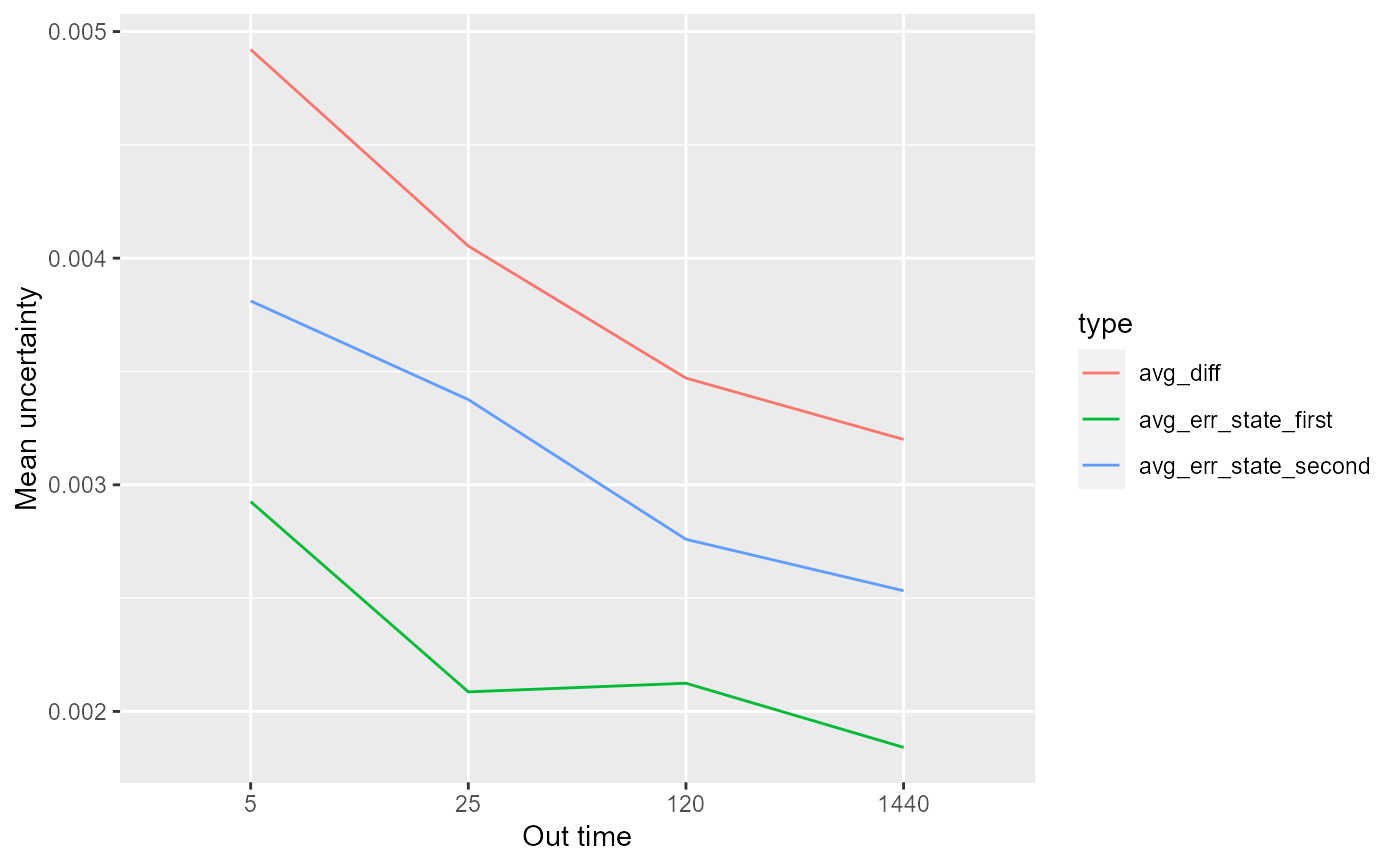Checks how the uncertainty changes in a function of out_time.

quality_control(dat, state_first, state_second, chosen_time, in_time)

## Arguments

dat data read by read_hdx state of the first peptide state of the second peptide chosen time point in time

## Value

data.frame with mean uncertainty per different out_time value

## Details

The function calculates mean uncertainty of all peptides and its uncertainty (standard error) based on given in_time and chosen_time as a function of out_time. Both theoretical and experimental results for each state and their difference are supplied for comparison but only experimental calculations depends on out_time variable. The results are either in form of relative or absolute values depending on the relative parameter supplied by the user. This data can be useful for general overview of the experiment and analyse of the chosen time parameters.

read_hdx

## Examples

# load example data

# calculate mean uncertainty
(result <- quality_control(dat = dat,
state_first = "CD160",
state_second = "CD160_HVEM",
chosen_time = 1,
in_time = 0.001))#>   out_time avg_err_state_first sd_err_state_first avg_err_state_second
#> 1        5         0.002925674        0.002207625          0.003811229
#> 2       25         0.002086275        0.001636480          0.003375989
#> 3      120         0.002124354        0.001284390          0.002759393
#> 4     1440         0.001841383        0.001032972          0.002532581
#>   sd_err_state_second    avg_diff     sd_diff
#> 1         0.004561764 0.004920756 0.004952401
#> 2         0.003690424 0.004053767 0.003949308
#> 3         0.001976257 0.003470818 0.002088545
#> 4         0.001120593 0.003200211 0.001369362
library(ggplot2)
library(tidyr)#>
#> Dołączanie pakietu: 'tidyr'#> Następujący obiekt został zakryty z 'package:testthat':
#>
#>     matcheslibrary(dplyr)

# example of data visualization
gather(result, 2:7, key = 'type', value = 'value') %>%
filter(startsWith(type, "avg")) %>%
ggplot(aes(x = factor(out_time), y = value, group = type)) +
geom_line(aes(color = type)) +
labs(x = "Out time",
y = "Mean uncertainty")